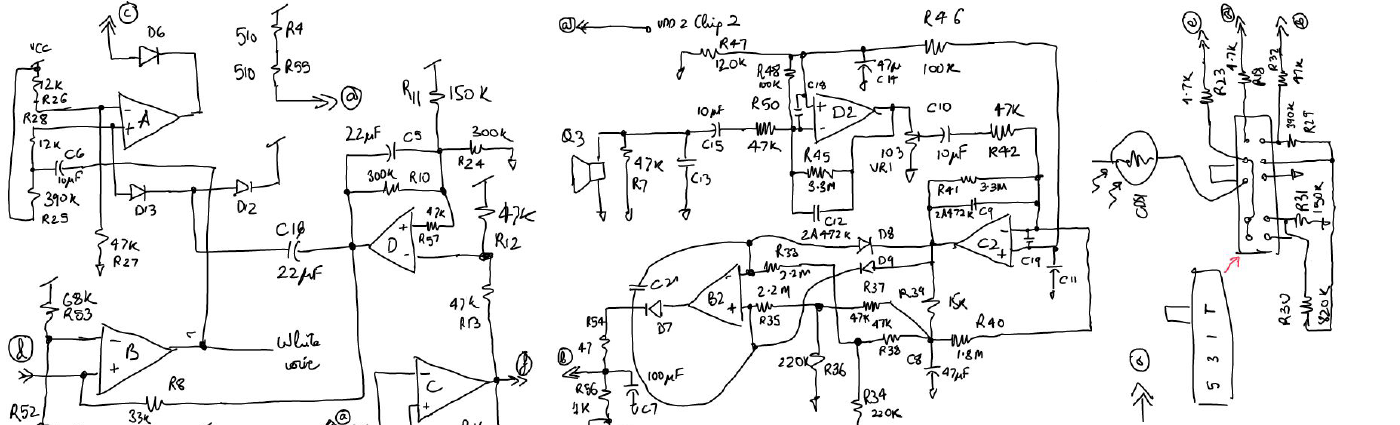# Minimizing RC area for given Time Constant# Objective [top]

During IC design it is often required to choose appropriate resistor and capacitor sizes to implement a filter or design networks. Here we derive a formula to decide what resistor and capacitor values can be used for a given time constant so that the area is minimized.

# Derivation [top]

`\$C_□\$` = capacitance per unit area for the process
`\$R_□\$` = resistance per square for the process
`\$τ\$` = Time constant of the circuit = `\$RC\$`
`\$\$Area=R/{R_□}W^2+C/{C_□}=τ/{CR_□}W^2+C/{C_□}\$\$`

Here W = width of the resistor, this is chosen based on the accuracy needed for the resistor according to the process data
Differentiating with respect to C we have:
`\$\${d{Area} }/{dC} = -τ/{R_□}W^2 1/{C^2}+1/{C_□}\$\$`

Equating that to 0 and calculating C we have:
`\$\$C=√{{τW^2C_□}/{R_□}}\$\$`

Thus using this capacitance value and using `\$R=τ/C\$` would give the optimized area for the RC network